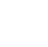Back to Chapter
Getting StartedBasic Syntax & DeclarationControl StatementsNo items found.
Function & EventsNo items found.
Array and its TypesNo items found.
OOP : Object Oriented ProgrammingNo items found.
Javascript StandardsNo items found.
HTML DOMNo items found.No items found.No items found.No items found.

# Return Functions

The Function that Gives Output According to The Input Provided. These are known as Return Function.

## Syntax

``` function functionName(parameters){ //statements return variableName; } var a = functionName(parameters); ```

The return statement can be used to return the value to a function call.

The return statement denotes that the function has ended. Any code after return is not executed.

## Example 1

First, We will make a program to add 2 numbers.

``` function add(num1,num2){ var total = num1 + num2 return total; } var sum = add(5,7); console.log(sum); //output //12 ```

Explanation :

All Parameters defined inside a ()  can be accessible inside Function Scope i.e. only inside { } Parenthesis.

## Example 2

Lets Assume, You have to convert Temperature (Fahrenheit to Celsius)

What you will do (Generally) :

Either you will create a variable and assign the value of Temperature in Fahrenheit

so, now we will use the 1st method.

``` var fahrenheit = 97; var celsius = (5/9) * (fahrenheit-32); console.log(celsius); //output //36.111111111111114 ```

Now, We will do the same task using the functions to make that function reusable and look our program clean.

``` function toCelsius(f) { return (5/9) * (f-32); } var fahrenheit = 97; console.log(toCelsius(fahrenheit)); //output //36.111111111111114 ```

Here, We have created a function with the name toCelsius( ) to convert Fahrenheit to celsius.

Whenever toCelsius(f) is called with Fahrenheit value as a parameter, it will output the converted celsius value using the return statement.

Then we can use returned value where ever we need. for example we have used the returned value to print in the console tab.